# Selina Concise Physics Class 10 ICSE Solutions Machines

## Selina Concise Physics Class 10 ICSE Solutions Machines

APlusTopper.com provides step by step solutions for Selina Concise ICSE Solutions for Class 10 Physics Chapter 3 Machines. You can download the Selina Concise Physics ICSE Solutions for Class 10 with Free PDF download option. Selina Publishers Concise Physics for Class 10 ICSE Solutions all questions are solved and explained by expert teachers as per ICSE board guidelines.

Selina ICSE Solutions for Class 10 Physics Chapter 3 Machines

Exercise 3(A)

Solution 1.

(a) A machine is a device by which we can either overcome a large resistive force at some point by applying a small force at a convenient point and in a desired direction or by which we can obtain a gain in the speed.

(b) An ideal machine is a machine whose parts are weightless and frictionless so that which there is no dissipation of energy in any manner. Its efficiency is 100%, i.e. the work output is equal to work input.

Solution 2.

Machines are useful to us in the following ways:

1. In lifting a heavy load by applying a less effort.
2. In changing the point of application of effort to a convenient point.
3. In changing the direction of effort to a convenient direction.
4. For obtaining a gain in speed.

Solution 3.

(a) To multiply force: a jack is used to lift a car.
(b) To change the point of application of force: the wheel of a cycle is rotated with the help of a chain by applying the force on the pedal.
(c) To change the direction of force: a single fixed pulley is used to lift a bucket full of water from the well by applying the effort in the downward direction instead of applying it upwards when the bucket is lifted up without the use of pulley.
(d) To obtain gain in speed: when a pair of scissors is used to cut the cloth, its blades move longer on cloth while its handles move a little.

Solution 4.

The purpose of jack is to make the effort less than the load so that it works as a force multiplier.

Solution 6.

The ratio of the load to the effort is called mechanical advantage of the machine. It has no unit.

Solution 7.

The ratio of the velocity of effort to the velocity of the load is called the velocity ratio of machine. It has no unit.

Solution 8.

For an ideal machine mechanical advantage is numerically equal to the velocity ratio.

Solution 9.

It is the ratio of the useful work done by the machine to the work put into the machine by the effort.
In actual machine there is always some loss of energy due to friction and weight of moving parts, thus the output energy is always less than the input energy.

Solution 10.

(a) A machine acts as a force multiplier when the effort arm is longer than the load arm. The mechanical advantage of such machines is greater than 1.
(b) A machine acts a speed multiplier when the effort arm is shorter than the load arm. The mechanical advantage of such machines is less than 1.

It is not possible for a machine to act as a force multiplier and speed multiplier simultaneously. This is because machines which are force multipliers cannot gain in speed and vice-versa.

Solution 11.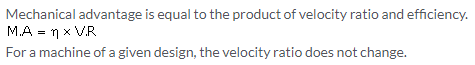Solution 12.

Let a machine overcome a load L by the application of an effort E. In time t, let the displacement of effort be dE and the displacement of load be dL.
Work input = Effort X displacement of effort = E X dE
Work output = Load X displacement of load = L X dL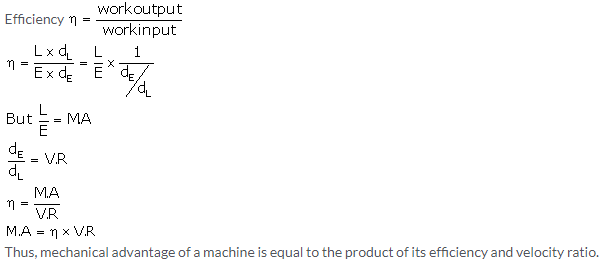Solution 13.The efficiency of such a machine is always less than 1, i.e. h<1. This is because there is always some loss in energy in form of friction etc.

Solution 14.

This is because the output work is always less than the input work, so the efficiency is always less than 1 because of energy loss due to friction.Solution 15.

A lever is a rigid, straight or bent bar which is capable of turning about a fixed axis.
Principle: A lever works on the principle of moments. For an ideal lever, it is assumed that the lever is weightless and frictionless. In the equilibrium position of the lever, by the principle of moments,

Solution 16.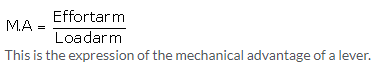Solution 17.

The three classes of levers are:

1. Class I levers: In these types of levers, the fulcrum F is in between the effort E and the load L. Example: a seesaw, a pair of scissors, crowbar.
2. Class II levers: In these types of levers, the load L is in between the effort E and the fulcrum F. The effort arm is thus always longer than the load arm. Example: a nut cracker, a bottle opener.
3. Class III levers: In these types of levers, the effort E is in between the fulcrum F and the load L and the effort arm is always smaller than the load arm. Example: sugar tongs, forearm used for lifting a load.

Solution 18.

(a) More than one: shears used for cutting the thin metal sheets.
(b) Less than one: a pair of scissors whose blades are longer than its handles.

Solution 19.

When the mechanical advantage is less than 1, the levers are used to obtain gain in speed. This implies that the displacement of load is more as compared to the displacement of effort.

Solution 20.

A pair of scissors and a pair of pliers both belong to class I lever.
A pair of scissors has mechanical advantage less than 1.

Solution 21.

A pair of scissors used to cut a piece of cloth has blades longer than the handles so that the blades move longer on the cloth than the movement at the handles.
While shears used for cutting metals have short blades and long handles because as it enables us to overcome large resistive force by a small effort.

Solution 22.

(a) The weight W of the scale is greater than E.
It is because arm on the side of effort E is 30 cm and on the side of weight of scale is 10 cm. So, to balance the scale, weight W of scale should be more than effort E.Solution 23.

Class II lever always have a mechanical advantage more than one.
Example: a nut cracker.
To increase its mechanical advantage we can increase the length of effort arm.

Solution 24.Solution 25.

In these types of levers, the load L is in between the effort E and the fulcrum F. So, the effort arm is thus always longer than the load arm. Therefore M.A > 1.

Solution 26.Solution 27.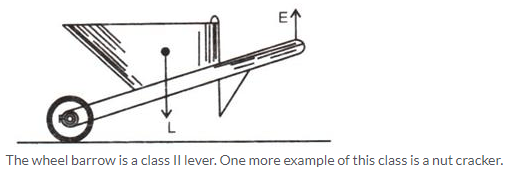Solution 29.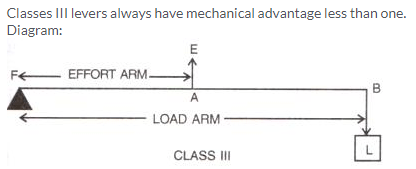Solution 30.

In these types of levers, the effort is in between the fulcrum F and the load L and so the effort arm is always smaller than the load arm. Therefore M.A. < 1.

Solution 31.

With levers of class III, we do not get gain in force, but we get gain in speed, that is a longer displacement of load is obtained by a smaller displacement of effort.

Solution 32.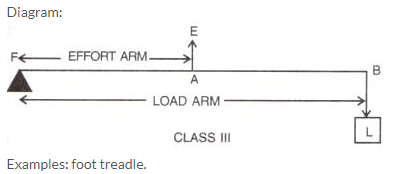Solution 33.

(a) A bottle opener is a lever of the second order, as the load is in the middle, fulcrum at one end and effort at the other.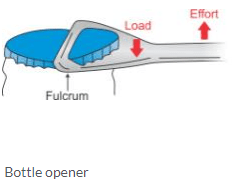(b) Sugar tongs is a lever of the third order as the effort is in the middle, load at one end and fulcrum at the other end.Solution 34.Solution 35.

a. Class II
b. Class I
c. Class II
d. Class III

Solution 36.

(a) Class III.
Here, the fulcrum is the elbow of the human arm. Biceps exert the effort in the middle and load on the palm is at the other end.(b) Class II.
Here, the fulcrum is at toes at one end, the load (i.e. weight of the body) is in the middle and effort by muscles is at the other end.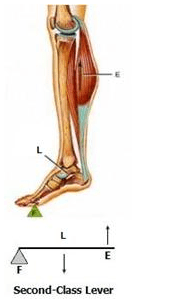Solution 37.Solution 38.

Class I lever in the action of nodding of the head: In this action, the spine acts as the fulcrum, load is at its front part, while effort is at its rear part.

Class II lever in raising the weight of the body on toes: The fulcrum is at toes at one end, the load is in the middle and effort by muscles is at the other end.

Class III lever in raising a load by forearm: The elbow joint acts as fulcrum at one end, biceps exerts the effort in the middle and a load on the palm is at the other end.

Solution 1 (MCQ).

M.A. x E = L

Solution 2 (MCQ).Solution 3 (MCQ).

It can have a mechanical advantage greater than the velocity ratio.
Reason: If the mechanical advantage of a machine is greater than its velocity ratio, then it would mean that the efficiency of a machine is more than 100%, which is practically not possible.

Solution 4 (MCQ).

Effort is between fulcrum and load
Hint: Levers, for which the mechanical advantage is less than 1, always have the effort arm shorter than the load arm.

Solution 5 (MCQ).

M.A > 1
Hint: In class II levers, the load is in between the effort and fulcrum. Thus, the effort arm is always longer than the load arm and less effort is needed to overcome a large load. Hence, M.A > 1.

Numericals

Solution 1.Solution 2.Solution 3.Solution 4.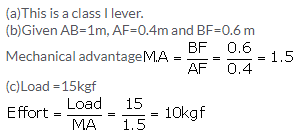Solution 5.Solution 6.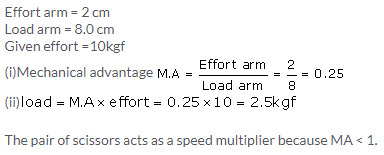Solution 7.

Total length of rod=4 m = 400 cm

(a) 18kgf load is placed at 60 cm from the support.
W kgf weight is placed at 250 cm from the support.
By the principle of moments
18 x 60 = W x 250
W = 4.32 kgf

(b) Given W=5 kgf
18kgf load is placed at 60 cm from the support.
Let 5 kgf of weight is placed at d cm from the support.
By the principle of moments
18 x 60 = 5 x d
d = 216 cm from the support on the longer arm

(c) It belongs to class I lever.

Solution 9.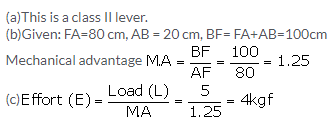Solution 10.Solution 11.

(a) The principle of moments: Moment of the load about the fulcrum=moment of the effort about the fulcrum
FB x Load = FA x Effort
(b) Sugar tongs the example of this class of lever.
(c) Given: FA=10 cm, AB = 500 cm, BF =500+10=510 cm.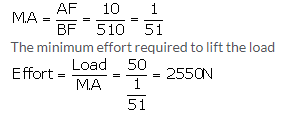Solution 12.Exercise 3(B)

Solution 1.

Fixed pulley: A pulley which has its axis of rotation fixed in position, is called a fixed pulley.
Single fixed pulley is used in lifting a small load like water bucket from the well.

Solution 2.

The ideal mechanical advantage of a single fixed pulley is 1.
It cannot be used as force multiplier.

Solution 3.

There is no gain in mechanical advantage in the case of a single fixed pulley. A single fixed pulley is used only to change the direction of the force applied that is with its use, the effort can be applied in a more convenient direction. To raise a load directly upwards is difficult.

Solution 4.

The velocity ratio of a single fixed pulley is 1.

Solution 5.

The load rises upwards with the same distance x.

Solution 6.

Single movable pulley: A pulley, whose axis of rotation is not fixed in position, is called a single movable pulley.
Mechanical advantage in the ideal case is 2.

Solution 8.

The efficiency of a single movable pulley system is not 100% this is because

1. The friction of the pulley bearing is not zero ,
2. The weight of the pulley and string is not zero.

Solution 9.

The force should be in upward direction.
The direction of force applied can be changed without altering its mechanical advantage by using a single movable pulley along with a single fixed pulley to change the direction of applied force.
Diagram: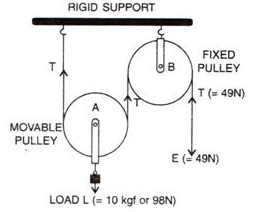Solution 10.

The velocity ratio of a single movable pulley is always 2.

Solution 11.

The load is raised to a height of x/2.

Solution 12.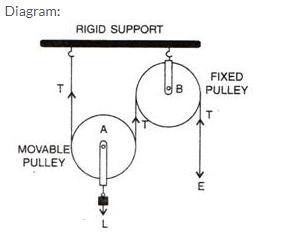Ideal mechanical advantage of this system is 2. This can be achieved by assuming that string and the pulley are massless and there is no friction in the pulley bearings or at the axle or between the string and surface of the rim of the pulley.

Solution 13.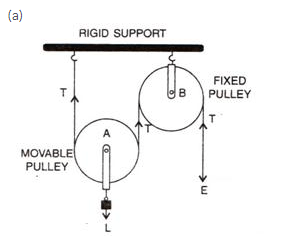(b) The fixed pulley B is used to change the direction of effort to be applied from upward to downward.
(c) The effort E balances the tension T at the free end, so E=T
(d) The velocity ratio of this arrangement is 2.
(e) The mechanical advantage is 2 for this system (if efficiency is 100%).

Solution 14.

 Single fixed pulley Single movable pulley 1. It is fixed to a rigid support. 1. It is not fixed to a rigid support. 2. Its mechanical advantage is one. 2. Its mechanical advantage istwo. 3. Its velocity ratio is one. 3. Its velocity ratio is two. 4. The weight of pulley itself does not affect its mechanical advantage. 4. The weight of pulley itself reduces its mechanical advantage. 5. It is used to change the direction of effort 5. It is used as force multiplier.

Solution 15.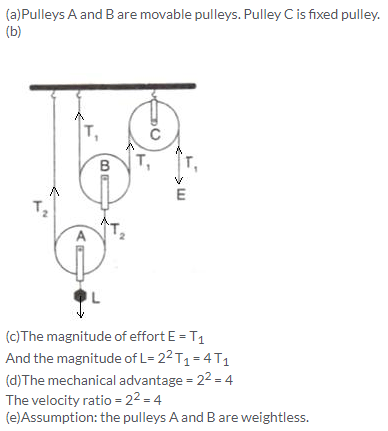Solution 16.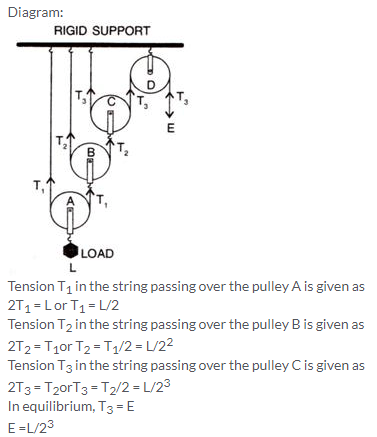Mechanical advantage = MA = L/E = 23
As one end of each string passing over a movable pulley is fixed, so the free end of string moves twice the distance moved by the movable pulley.
If load L moves up by a distance x, dL = x, effort moves by a distance 23x, dE = 23x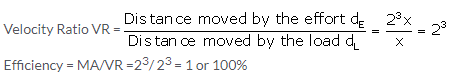Solution 17.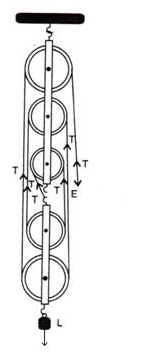Solution 18.

1. In a single fixed pulley, some effort is wasted in overcoming friction between the strings and the grooves of the pulley; so the effort needed is greater than the load and hence the mechanical advantage is less than the velocity ratio.
2. This is because of some effort is wasted in overcoming the friction between the strings and the grooves of the pulley.
3. This is because mechanical advantage is equal to the total number of pulleys in both the blocks.
4. The efficiency depends upon the mass of lower block; therefore efficiency is reduced due to the weight of the lower block of pulleys.

Solution 19.

(a) Multiply force: a movable pulley.
(b) Multiply speed: gear system or class III lever.
(c) Change the direction of force applied: single fixed pulley.

Solution 20.

1. The velocity ratio of a single fixed pulley is always more than 1.(false)
2. The velocity ratio of a single movable pulley is always 2.(true)
3. The velocity ratio of a combination of n movable pulleys with a fixed pulley is always 2n.(true)
4. The velocity ratio of a block and tackle system is always equal to the number of strands of the tackle supporting the load. (true)

Solution 1 (MCQ).

It helps in applying effort in a convenient direction.
Explanation: A single fixed pulley though does not reduce the effort but helps in changing the direction of effort applied. As it is far easier to apply effort in downward direction, the single fixed pulley is widely used.

Solution 2 (MCQ).

The mechanical advantage of an ideal single movable pulley is 2.
Derivation: Consider the diagram given below:Here the load L is balance by the tension in two segments of the string and the effort E balances the tension T at the free end, so
L = T + T = 2T and E = T
Assumption: Weight of the pulley is negligible.
We know that,Solution 3 (MCQ).

Force multiplier
Explanation: The mechanical advantage of movable pulley is greater than 1. Thus, using a single movable pulley, the load can be lifted by applying an effort equal to half the load (in ideal situation), i.e. the single movable pulley acts as a force multiplier.

Numericals

Solution 1.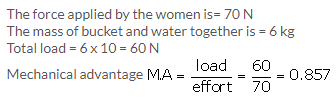Solution 2.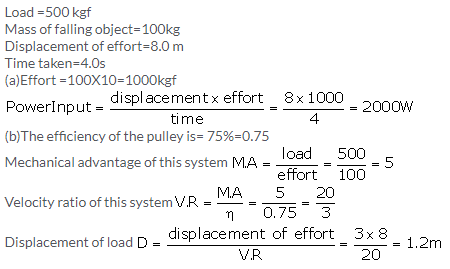Solution 3.Solution 4.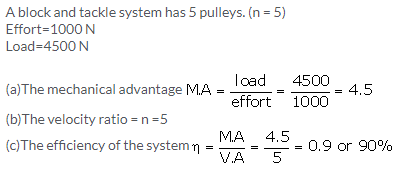Solution 5.Solution 6.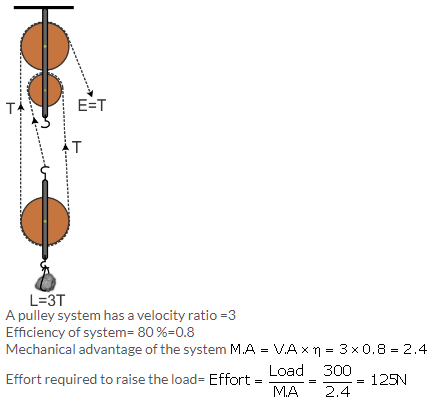Solution 7.(d) (i) There is no friction in the pulley bearings, (ii) weight of lower pulleys is negligible and (iii) the effort is applied downwards.

Solution 8.Solution 9.Solution 10.More Resources for Selina Concise Class 10 ICSE Solutions